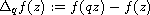Electron. J. Differential Equations, Vol. 2017 (2017), No. 175, pp. 1-12.

### Some properties of meromorphic solutions for q-difference equations Hong Yan Xu, San Yang Liu, Xiu Min Zheng

Abstract:
The main purpose of this article is to investigate some properties on the meromorphic solutions of some types of q-difference equations, which can be seen the q-difference analogues of Paineve equations. We obtain estimates of the exponent of convergence of poles of, which extends some earlier results by Chen et al.

Submitted February 14, 2017. Published July 10, 2017.
Math Subject Classifications: 39A50, 30D35
Key Words: Meromorphic function; q-difference equation; zero order.

Show me the PDF file (226 KB), TEX file for this article.Hong Yan Xu School of mathematics and statistics Xidian University Xi'an, Shaanxi 710126, China email: xhyhhh@126.com} San Yang Liu School of mathematics and statistics Xidian University Xi'an, Shaanxi 710126, China email: liusanyang@126.com Xiu Min Zheng Department of Mathematics Jiangxi Normal University Nanchan, Jiangxi 330022, China email: zhengxiumin2008@sina.com